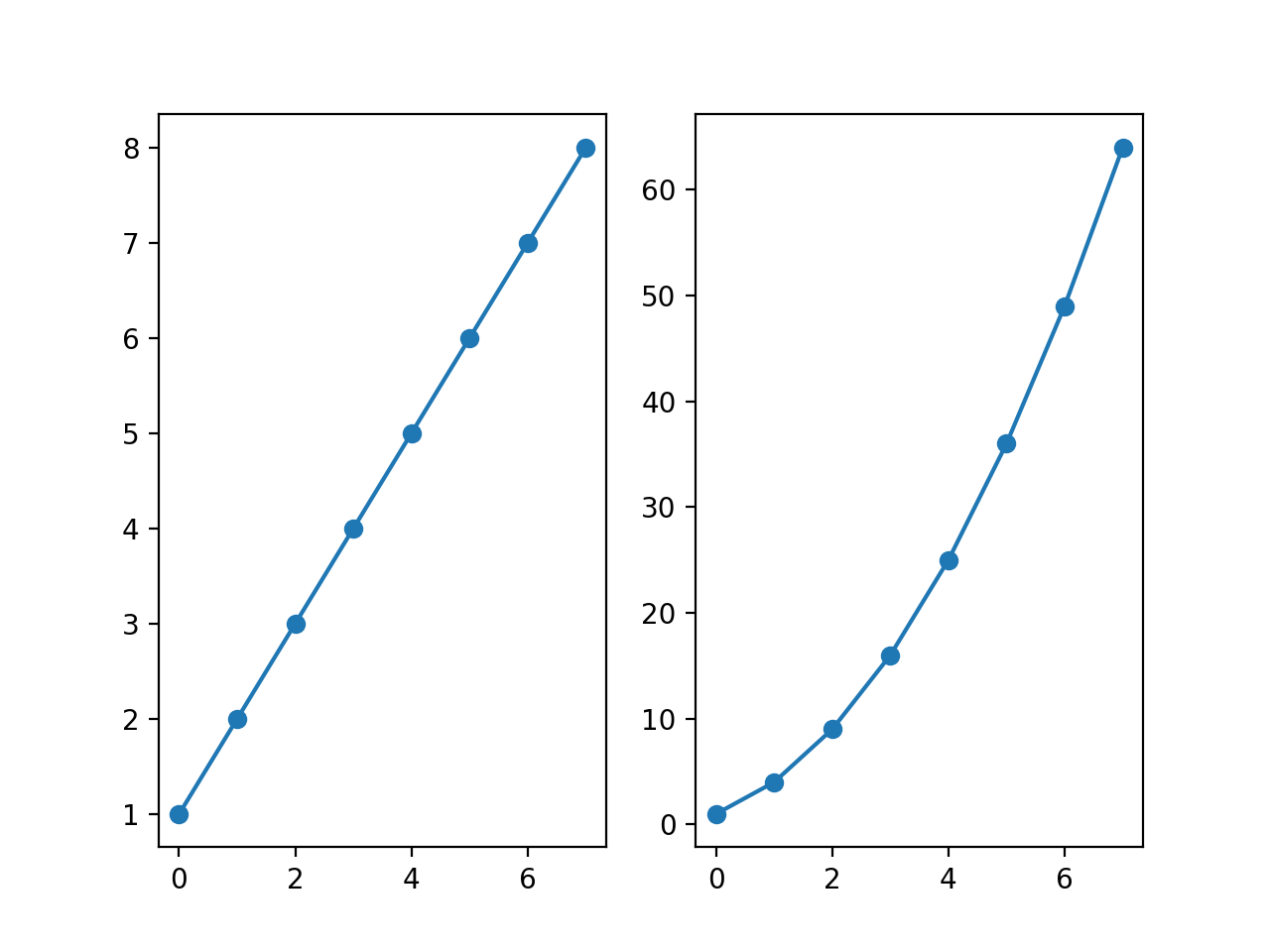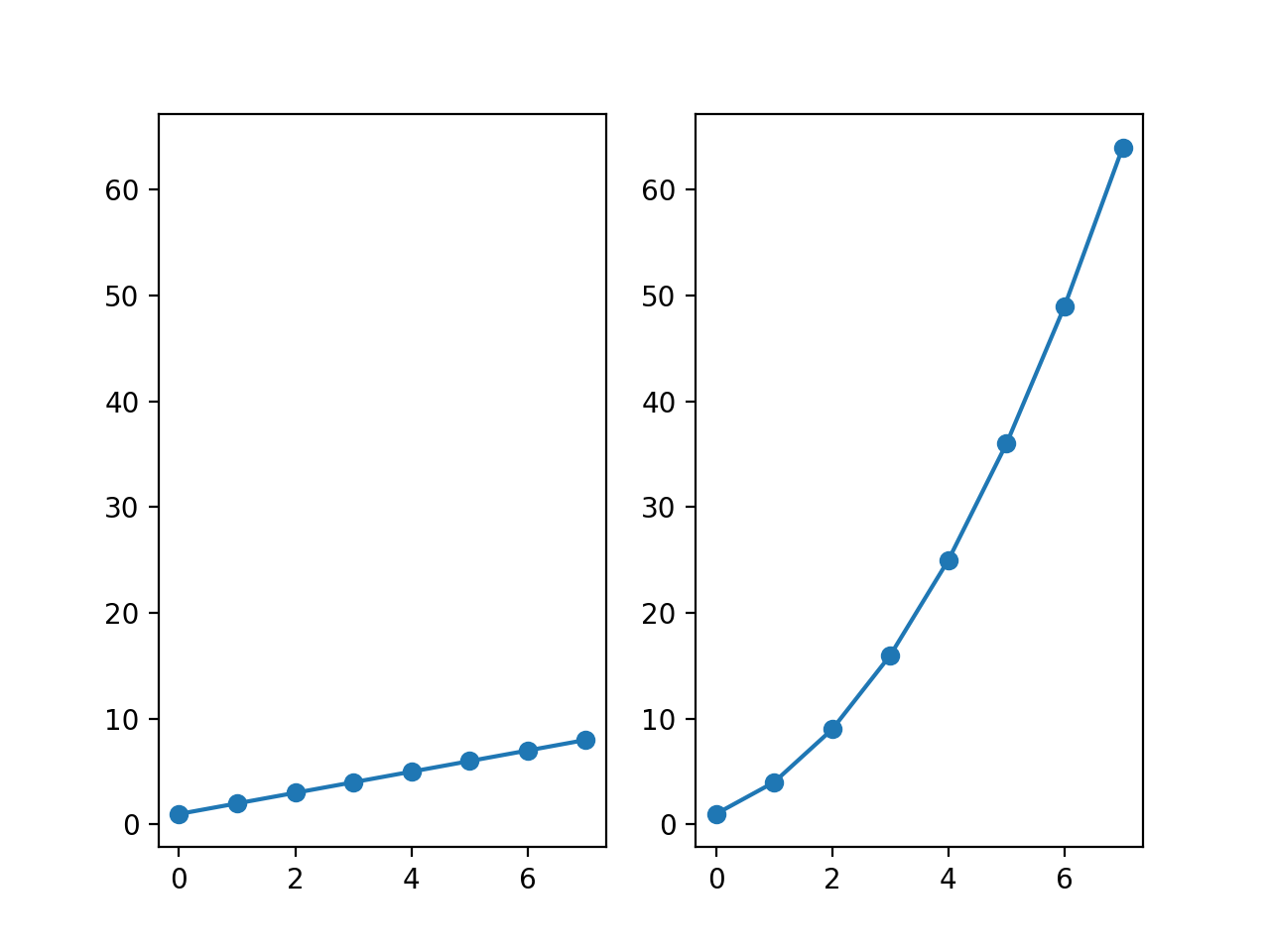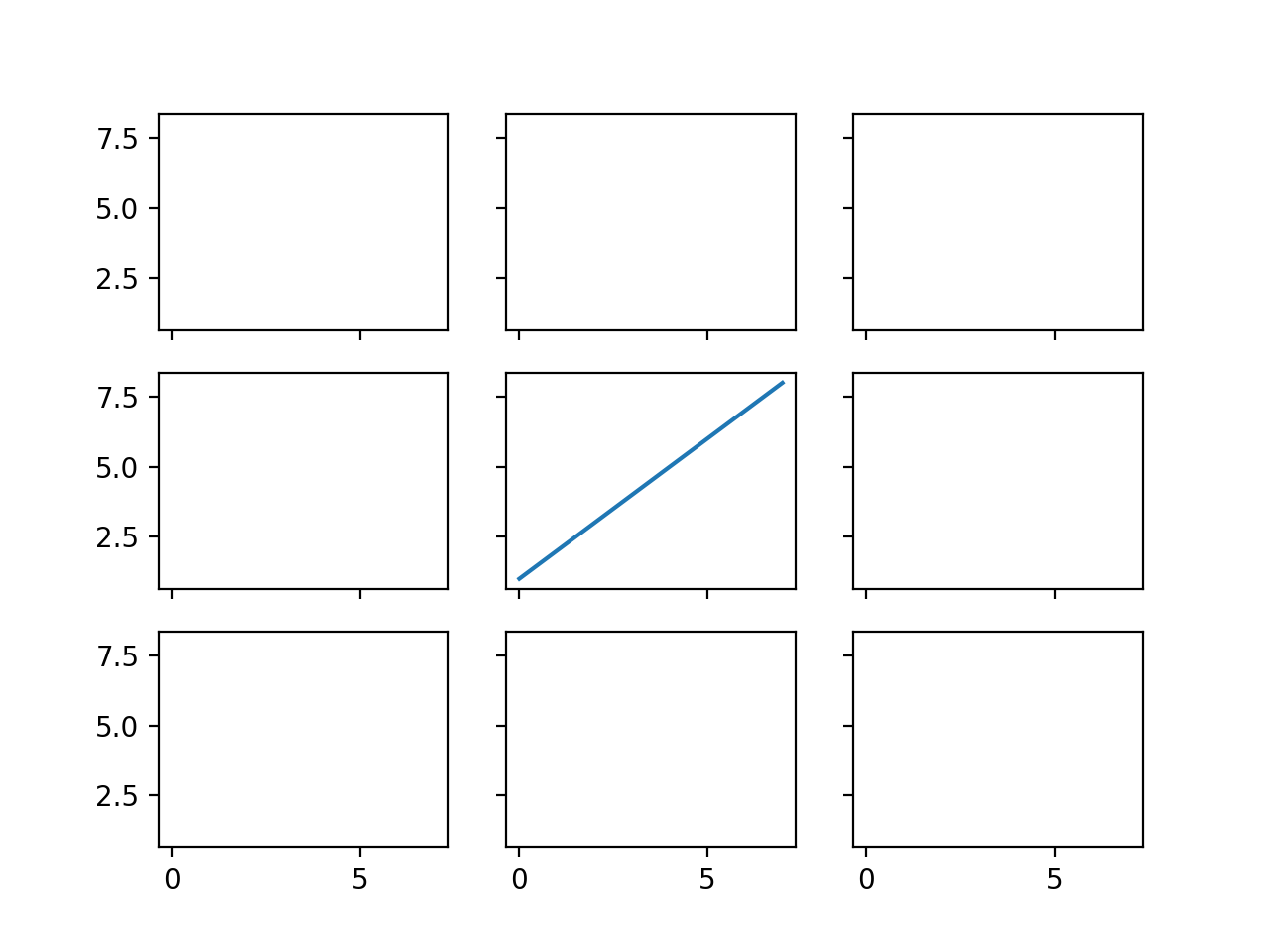# Subplots

Sometimes it is useful to show two complex plots side by side for a veiwer to compare. Matplotlib handles this situation with a single figure that has two plots on it.

The subplot command allows you to create axes on different portions of a grid that each figure contains.

%matplotlib notebook

import matplotlib.pyplot as plt
import numpy as np


The following command creates a subplot with 1 row, 2 columns, and sets the current axis to the first subplot axes.

plt.figure()
plt.subplot(1, 2, 1)

linear_data = np.array([1,2,3,4,5,6,7,8])

plt.plot(linear_data, '-o');

<IPython.core.display.Javascript object>The following sets the axis to the second subplot axes and then plots exponential data on it. It uses the same figure as shown above.

Note that the third parameter, which sets which subplot will be used, is 1-indexed and not 0-indexed.

exponential_data = linear_data**2

plt.subplot(1, 2, 2)
plt.plot(exponential_data, '-o');


Note that pyplot does not automatically lock axes between the two plots.

The following illustrates how to lock the y-axes of two subplots.

plt.figure()

ax1 = plt.subplot(1, 2, 1)
plt.plot(linear_data, '-o')

ax2 = plt.subplot(1, 2, 2, sharey=ax1)
plt.plot(exponential_data, '-o');

<IPython.core.display.Javascript object>The subplots command can also create larger grids of axes.

It is an effective way to create large grids where everything has locked axes.

fig, ((ax1,ax2,ax3),
(ax4,ax5,ax6),
(ax7,ax8,ax9)) = plt.subplots(3, 3,
sharex=True,
sharey=True)

ax5.plot(linear_data, '-');

<IPython.core.display.Javascript object>